Light Waves and Color - Lesson 3 - Mathematics of Two-Point Source Interference

The Path Difference

Two-point source interference occurs when waves from one source meet up with waves from another source. If the source of waves produces circular waves, then the circular wavefronts will meet within the medium to produce a pattern. The pattern is characterized by a collection of nodes and antinodes that lie along nearly straight lines referred to as antinodal lines and nodal lines. If the wave sources have identical frequencies, then there will be an antinodal line in the exact center of the pattern and an alternating series of nodal and antinodal lines to the left and the right of the central antinodal line. As discussed in the previous section of Lesson 3, each line in the pattern is assigned a name (e.g., first antinodal line) and an order number (represented by the symbol m). A representative two-point source interference pattern with accompanying order numbers (m values) is shown below.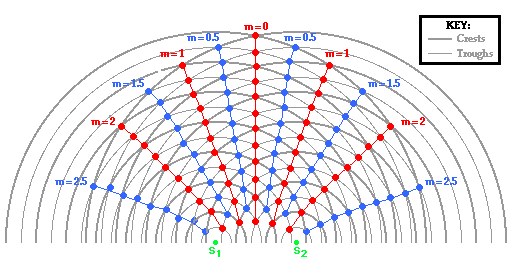In this part of Lesson 3, we will investigate the rationale behind the numbering system and develop some mathematical equations that relate the features of the pattern to the wavelength of the waves. This investigation will involve the analysis of several antinodal and nodal locations on a typical two-point source interference pattern. It will be assumed in the discussion that the wave sources are producing waves with identical frequencies (and therefore identical wavelengths).

To begin, consider the pattern shown in the animation below. Point A is a point located on the first antinodal line. This specific antinode is formed as the result of the interference of a crest from Source 1 (S1) meeting up with a crest from Source 2 (S2). The two wave crests are taking two different paths to the same location to constructively interfere to form the antinodal point.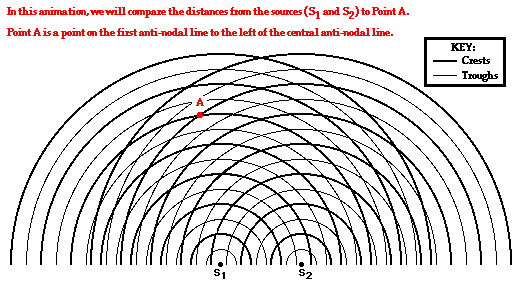The crest traveling from Source 1 (S1) travels a distance equivalent to 5 full waves; that is, point A is a distance of 5 wavelengths from Source 1 (S1). The crest traveling from Source 2 (S2) travels a distance equivalent to 6 full waves; point A is a distance of 6 wavelengths from Source 2 (S2). While the two wave crests are traveling a different distance from their sources, they meet at point A in such a way that a crest meets a crest. For this specific location on the pattern, the difference in distance traveled (known as the path difference and abbreviated as PD) is

PD = | S1A - S2A | = | 5λ - 6λ | = 1λ

(Note the path difference or PD is the difference in distance traveled by the two waves from their respective sources to a given point on the pattern.)

For point A on the first antinodal line (m =1), the path difference is equivalent to 1 wavelength. But will all points on the first antinodal line have a path difference equivalent to 1 wavelength? And if all points on the first antinodal line have a path difference of 1 wavelength, then will all points on the second antinodal line have a path difference of 2 wavelengths? And what about the third antinodal line? And what about the nodal lines? These questions are investigated in the diagrams below through the analysis of the path difference for other points located on antinodal and nodal lines.

Point B in the diagram below is also located on the first antinodal line. The point is formed as a wave crest travels a distance of 3 wavelengths from point S1 and meets with a second wave crest that travels a distance 4 wavelengths from S2. The difference in distance traveled by the two waves from their sources to point B is

PD = | S1B - S2B | = | 3λ - 4λ | = 1λ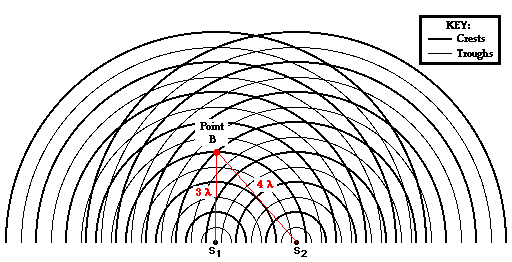Now we will analyze Point C, which is located on the second antinodal line (see the diagram below). The point is formed as a wave crest travels a distance of 4 wavelengths from point S1 and meets with a second wave crest that travels a distance 6 wavelengths from S2. The difference in distance traveled by the two waves from their sources to point C is

PD = | S1C - S2C | = | 4 λ - 6 λ | = 2 λ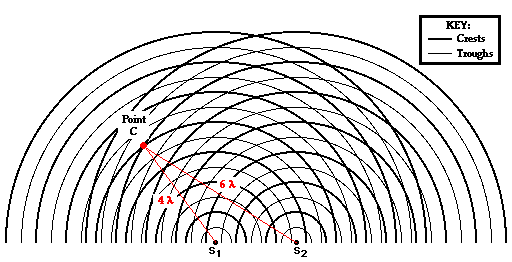The analysis continues for this same pattern as we explore the path difference for locations on nodal lines. Point D is located on the first nodal line (see the diagram below). The point is formed as a wave crest travels a distance of 5 wavelengths from point S1 and meets with a wave trough that travels a distance 4.5 wavelengths from S2. The difference in distance traveled by the two waves from their sources to point D is

PD = | S1D - S2D | = | 5 λ - 4.5 λ | = 0.5 λ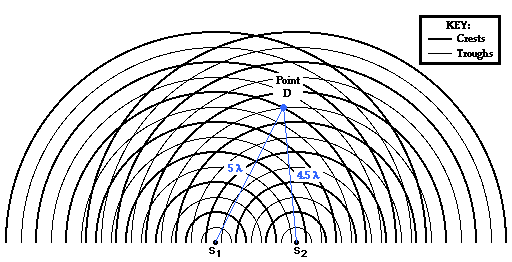Point E in the diagram below is located on the second nodal line. The point is formed as a wave trough travels a distance of 3.5 wavelengths from point S1 and meets with a wave crest that travels a distance 5 wavelengths from S2. The difference in distance traveled by the two waves from their sources to point E is

PD = | S1E - S2E | = | 3.5 λ - 5 λ | = 1.5 λ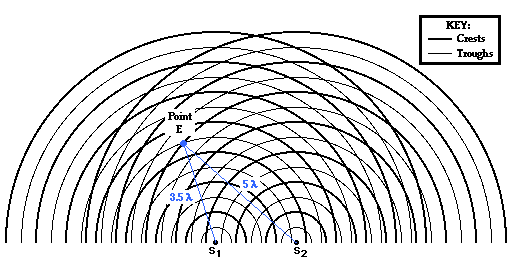The information in the above analyses is summarized in the table below. Other points on other antinodal and nodal lines are marked on the diagram below and their distance from the sources and their path difference are also summarized in the same table. Inspect the table and see if you can find a pattern evident in the numbers.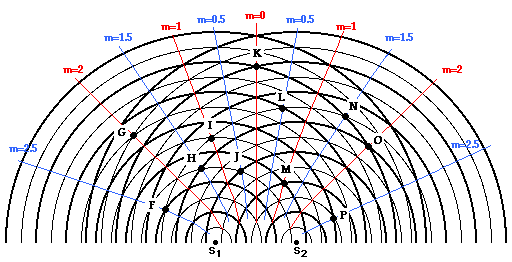Summary of the Path Difference Analysis

 Point Antinode or Node? Order # (m) Distance from S1 (in λ) Distance from S2 (in λ) Path Difference (in λ) A Antinode 1 5 λ 6 λ 1 λ B Antinode 1 3 λ 4 λ 1 λ C Antinode 2 4 λ 6 λ 2 λ D Node 0.5 5 λ 4.5 λ 0.5 λ E Node 1.5 3.5 λ 5 λ 1.5 λ F Node 2.5 2 λ 4.5 λ 2.5 λ G Antinode 2 4.5 λ 6.5 λ 2 λ H Node 1.5 2.5 λ 4 λ 1.5 λ I Antinode 1 3.5 λ 4.5 λ 1 λ J Node 0.5 2.5 λ 3 λ 0.5 λ K Antinode 0 6 λ 6 λ 0 λ L Node 0.5 5 λ 4.5 λ 0.5 λ M Antinode 1 3 λ 2 λ 1 λ N Node 1.5 6 λ 4.5 λ 1.5 λ O Antinode 2 6 λ 4 λ 2 λ P Node 2.5 4 λ 1.5 λ 2.5 λ

What pattern do you see in the numerical values for path difference above? An inspection of the path difference column and the order number column reveals that there is a clear relationship between these two quantities. The path difference is always the order number multiplied by the wavelength. That is,

PD = m • λ

Furthermore, one might notice that the path difference is a whole number of wavelengths for the antinodal positions and a half number of wavelengths for the nodal positions. That is,

 Antinodal Points: PD = m • λ where m = 0, 1, 2, 3, 4, ... Nodal Points: PD = m • λ where m = 0.5, 1.5, 2.5, 3.5, ...

Explaining the Path Difference Equation

A tedious inspection of a variety of antinodal and nodal points on a typical pattern reveals the above relationships. But why? Why would constructive interference occur when the difference in distance traveled by two waves is equivalent to a whole number of wavelengths? And why would destructive interference occur when the difference in distance traveled by two waves is equivalent to a half number of wavelengths? To answer these questions, consider the diagrams below.

The diagram below shows two waves traveling along different paths from different sources to the same point in such a way that a crest is meeting a crest. Constructive interference will occur at this point. The wave from Source 1 (S1) travels a distance of 6 wavelengths (you can trace your finger over the wave to help you count wavelengths). The wave from Source 2 (S2) travels a distance of 7 wavelengths to reach the same point. The difference in distance traveled by the two waves is one full wavelength. That is, the path difference is 1 λ. When the path difference is one full wavelength, a crest meets a crest and constructive interference occurs.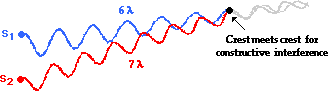How does a path difference of two wavelengths cause constructive interference? The diagram below shows this. Two waves traveling along different paths from different sources meet at the same point in such a way that a crest is meeting a crest; constructive interference occurs. The wave from Source 1 (S1) travels a distance of 6 wavelengths. The wave from Source 2 (S2) travels a distance of 8 wavelengths to reach the same point. The difference in distance traveled by the two waves is two full wavelengths; that is, the path difference is 2 λ. When the path difference is two full wavelengths, a crest meets a crest and constructive interference occurs.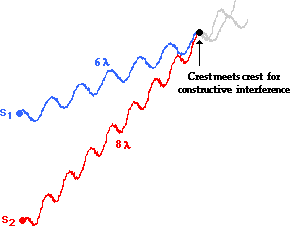The previous two examples involve the meeting of a crest with a crest. Under what conditions will a trough meet a trough? The diagram below depicts this. Once more, the two waves travel different paths from their different sources and meet in such a manner to constructively interfere; only this time the constructive interference results from a trough meeting a trough. Observe that the wave from Source 1 (S1) travels a distance of 6.5 wavelengths. The wave from Source 2 (S2) travels a distance of 7.5 wavelengths to reach the same point. The difference in distance traveled by the two waves is one full wavelength; that is, the path difference is 1 λ.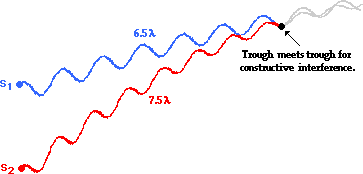The above examples pertain to the constructive interference that occurs for locations on antinodal lines. In each case, a path difference of a whole number of wavelengths causes a crest to meet a crest or a trough to meet a trough. But what about destructive interference? The diagram below depicts the destructive interference of two waves from the sources. Observe that the wave from Source 1 (S1) travels a distance of 3.5 wavelengths. The wave from Source 2 (S2) travels a distance of 4 wavelengths to reach the same point. The difference in distance traveled by the two waves is one-half a wavelength; that is, the path difference is 0.5 λ. Whenever the two waves have a path difference of one-half a wavelength, a crest from one source will meet a trough from the other source. Destructive interference occurs for path differences of one-half a wavelength.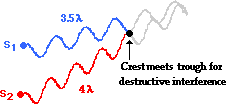An additional example of destructive interference is shown below. Observe that the wave from Source 1 (S1) travels a distance of 4.5 wavelengths. The wave from Source 2 (S2) travels a distance of 6 wavelengths to reach the same point. The difference in distance traveled by the two waves is three-halves a wavelength; that is, the path difference is 1.5. Whenever the two waves have a path difference of 1.5 wavelengths, a crest from one source will meet a trough from the other source and destructive interference will occur.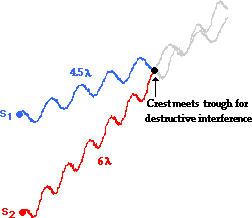In summary, the analysis of the two-point source interference patterns reveals the following equations:

 Antinodal Points: PD = m • λ where m = 0, 1, 2, 3, 4, ... Nodal Points: PD = m • λ where m = 0.5, 1.5, 2.5, 3.5, ...

A simple wave model demonstrates why these relationships exist.

In the next part of Lesson 3 we will investigate the nature of a two-point source light interference and make the connection between these simple ripple tank patterns and the observations made by Thomas Young when he first demonstrated two-point source light interference in the early 1800s.

1. Two point sources, 3.0 cm apart, are generating periodic waves in phase. A point on the third antinodal line of the wave pattern is 10 cm from one source and 8.0 cm from the other source. Construct a sketch of the physical situation and determine the wavelength of the waves. PSYW

2. Two point sources are generating periodic waves in phase. The wavelength is 4.0 cm. A point on the second antinodal line is 30.0 cm from the nearest source. How far is this point from the farthest source? Begin by constructing a sketch of the physical situation. PSYW

3. Two point sources are generating periodic waves in phase. The wavelength of the waves is 3.0 cm. A point on a nodal line is 25 cm from one source and 20.5 cm from the other source. Construct a sketch of the physical situation and determine the nodal line number. PSYW

4. Two point sources are generating periodic waves in phase. A point on the fourth nodal line is 25.0 cm from one source and 39.0 cm from the farthest source. Construct a sketch of the physical situation and determine the wavelength. PSYW

Next Section: